The sphere-cone equation in a matrix notation.

It is about time for a new post on 3D numbers, circular and complex. In this post I write the sphere-cone equation in a matrix notation so see the previous post on conjugates if you feel confused. The sphere-cone equation gives us two equations, as the name suggests these are a sphere and a cone and on the intersection we find the famous exponential circle.

Beside the sphere-cone equation I also demand that the determinant equals 1, now we have three equations and every intersection of those 3 equations has as it’s solution the exponential circle. Can it become more crazy? Yes because it is possible to factorize the third degree determinant into a linear and a quadratic factor. Those factors must also be 1 and now we have five equations! And since you can pick 10 pairs out of five, we now have 10 ways of solving for the intersection where the exponential circle lives…

It is strange that after all these years it is still easy to find 10 video’s where so called ‘professional math professors’ sing their praise upon the exponential circle in the complex plane. They really go beserk over the fact that e to the power it gives the cosine and sine thing. And after all those years still silent, yeah yeah those hero’s really deserve the title of honorable shithole… It is honorable because they often have relatively large salaries and they are shitholes because of their brave behavior when it comes to 3D complex numbers. Bah, I am getting a bad taste in my mouth when I think about the behavior of professional math professors. Let me stop writing about that low form of life.

This post is 8 pictures long. May be, I have not decided yet, is the next post about parametrizations of the exponential circle. In these 8 pictures I work out the case for the circular multiplication, that is the case where the imaginary unit j behaves like j^3 = 1. At the end I only give the 3D complex version of the matrix form of the sphere-cone equation and the rest you are supposed to do yourself.

Ok, again do not confuse this with quadratic forms. A matrix equation as written above has a real and two imaginary components while quadratic forms are often just real valued.

Let´s try to upload this stuff. See you in the next post.

But are these quadratic forms?

This is a lazy easy going summer post, it does not have much mathematical depth. Let’s say the depth of a bird bath. But with most posts I write you also need a lot of knowledge about what was in previous posts and for the average person coming along that is often too time consuming… So we keep it simple today; quadratic forms on 3D space.

If you have had one or two courses of linear algebra you likely have encountered quadratic forms. They are often denoted as Q(X) where the X is a column matrix and the quadratic form is defined as Q(X) = XT A X. Here XT is the transponent of X so that would be a matrix row. As you might guess, the X column matrix contains the variables while the constant square matrix A is the source of coefficients in the quadratic form Q(X).  In most literature it is told the matrix A is symmetric, of course there is no reason at all for that; any square matrix will do. On the other hand it is easy to see or to show that if a square matrix is anti-symmetric the corresponding quadratic form will always be zero everywhere.

In this post we will take matrices that are always the matrix representation of 3D complex & circular numbers. Matrix representations are a complete category on this website so if you don’t know them you must look that up first. (Oh oh, here I go again: this was supposed to be easy but now the average reader must first try to understand matrix representations of higher dimensional multiplications…)

Compared to the previous update on the likely failure of all fusion reactors this post is far less dramatic. If in the future I am right and we will never have fusion power, that will be the difference between life and death of hundreds of millions of people in the long run… So in order to be a bit less depressing let’s lift the spirits by a lightweight new post on quadratic forms! Why not enjoy life as long as it lasts?

Ok, the actual post is seven pictures long, all in the usual size of 550×775 pixels.

I have to admit that for me the use of the number alpha was important because that is at the center of the exponential circles in the 3D complex and circular spaces. So I have a legitimate reason to post this also under the category ´exponential circle´. And from the non-bird bath deep math, that is the big math ocean that is very deep, I like to classify as much posts under that category ´exponential circles´.

Ok, let´s leave it with that and try to upload this post. Till updates my dear reader.

New roots of unity (the 3D complex ones) & The rain theorem.

I just finished brewing the 100-th batch of a beer under the names Dark Matter and Spin 1/2 beer. All in all that is an amazing amount of beer; in the past I brewed 35 liter per batch but now it is 40 liter per batch so all in all an amount of something between 3500 and 4000 liters… So ein prosit my dear reader.

The ‘new’ roots of unity aren’t that new, this post is a re-editing of something I posted on 05 Jan 2014 on the other website. Later that year I started this website. Actually these roots of unity are just over five years old. In mathematical terms that is still very young so in that sense they are still new.

Recall the roots of unity in the complex plane are solutions to z^n = 1 and as such these roots are found on the exponential circle (the complex exponential) in the complex plane. As you have found the exponential circle or exponential curve in some space, from that you can always make new roots of unity. That is hardly a mathematical achievement because it is so simple to do once you have found your exponential circle or curve. But in the diverse spaces these new roots of unity behave very different, for example in this post we will add them all up but unlike in the complex plane they do not add up to zero. That is caused by the fact that in the complex 3D space the number alpha is at the center of the exponential circle, as such if we add n roots of unity in 3D space the result is n times alpha. Last year we studied the space of 4D complex numbers and if you would craft new roots of unity in that space it will behave much more like those in the complex plane because in the 4D complex numbers we have 0 as the center of the exponential curve. (For dimensions above 3 the exponential curve always lies in a hyperplane so it can never be a circle.) It always amazes me that you have all those physics people who study string theory but as far as I know never use exponential curves…

Life is beautiful, because how can you do string theory without math like that? But in physics almost everything is beautiful, for example if they explain the outcome of the Stern-Gerlach experiment always 50% of unpaired electrons align with the applied magnetic field and the other 50% for some mysterious reason do the anti-align thing. And if one hour later the same physics professor explains how a permanent magnet can attract some piece of iron, all of a sudden 100% of the unpaired electrons align and all that talk of 50/50 suddenly is not observed… Life inside the science of physics is wonderful; all you have to do is a bit of blah blah blah and if people complain this is not logical at all you simply say: Quantum physics is such that if you think you understand it, you don’t understand it… How wonderful is the life of physics professors; talk some blah blah blah and if people complain you blame them for ‘not understanding quantum things’. For sure that is a beautiful form of life.

But enough of the talking, somewhere in the next seven pictures I did forget to insert a graph of the determinant. Yet I showed you the structure of the non-invertible numbers so often, I think I post it with that fault included. After all why should life be perfect? If life would be perfect you would have no way of improvement and likely that is the moment you die: no more possibility of improvement. As usual the pictures are 550×775 pixels but I had to make the first one a tiny bit longer. Good luck with digesting it & have a bit of fun in the process.

For myself speaking it was fun to read my own two proof for the rain theorem again after five years. Please do not forget that new roots of unity on other spaces can be very different in behavior, after all they are always part of the exponential circle or curve in that space so they will derive their math properties from that. Till updates.

Part 19: Four integrals defining the 4D complex number tau.

It is a bit late but a happy new year anyway! In this post we will do a classic from the complex plane: calculation of the log of the first imaginary unit.

On the complex plane this is log i and on the complex 4D space this is log l .

Because this number is so important I have given it a separate name a long long time ago: These are the numbers tau in the diverse dimensions. In the complex plane it has no special name and it simply is i times pi/2.

On the real line it is pretty standard to define the log functions as the integral of the inverse 1/x. After all the derivative of log x on the real line is 1/x and as such you simply define the log to be the integral of the derivative…

On the complex plane you can do the same but depending of how your path goes around zero you can get different answers. Also in the complex plane (and other higher dimensional number systems) the log is ‘multi valued’. That is a reflection of the fact we can find exponential periodic functions also known as the exponential circles and curves.

The integrals in this part number 19 on the basics of 4D complex numbers are very hard to crack. I know of no way to find primitives and to crack them that way. May be that is possible, may be it is not, I just do not know. But because I developed the method of matrix diagonals for finding expressions for the value of those difficult looking integrals, more or less in an implicit manner we give the right valuations to those four integrals.

With the word ‘implicit’ I simply mean we skip the whole thing of caculating the number tau via matrix diagonalization. We only calculate what those integrals actually are in terms of a half circle with coordinates cos t and sin t.

This post is 8 pictures long in the usual size of 550 by 775 pixels (I had to enlarge the latest picture a little bit). I hope it is not loaded with typo’s any more and you have a more or less clean mathematical experience: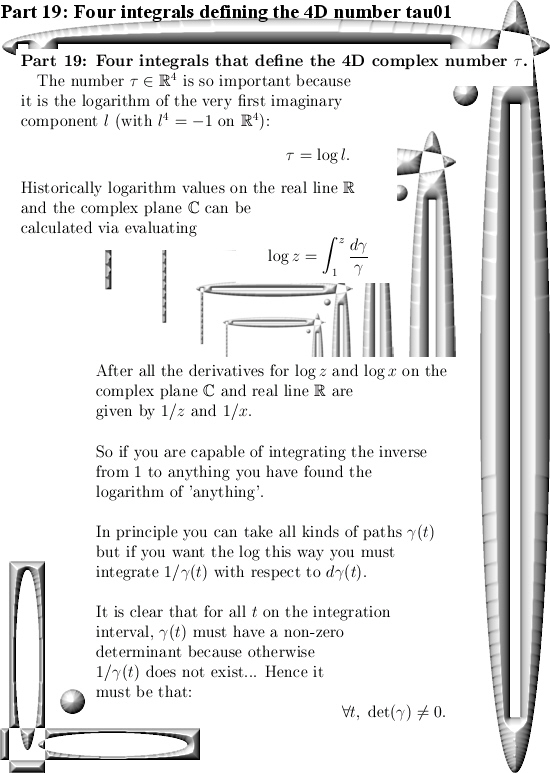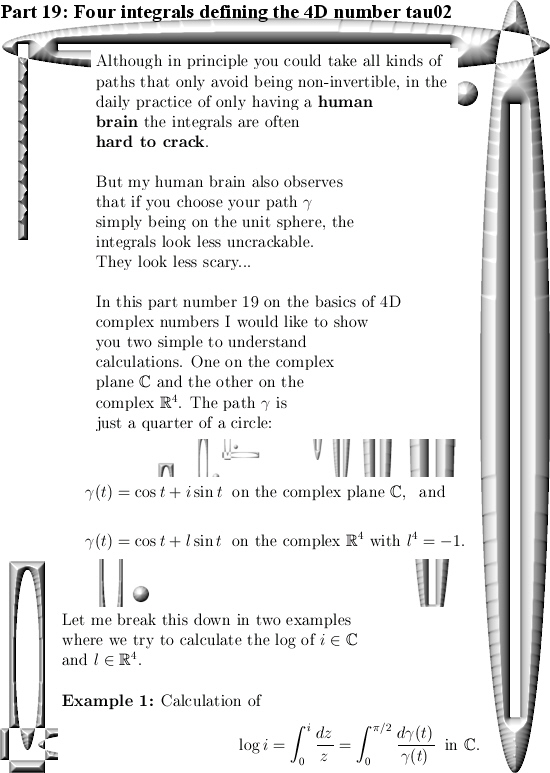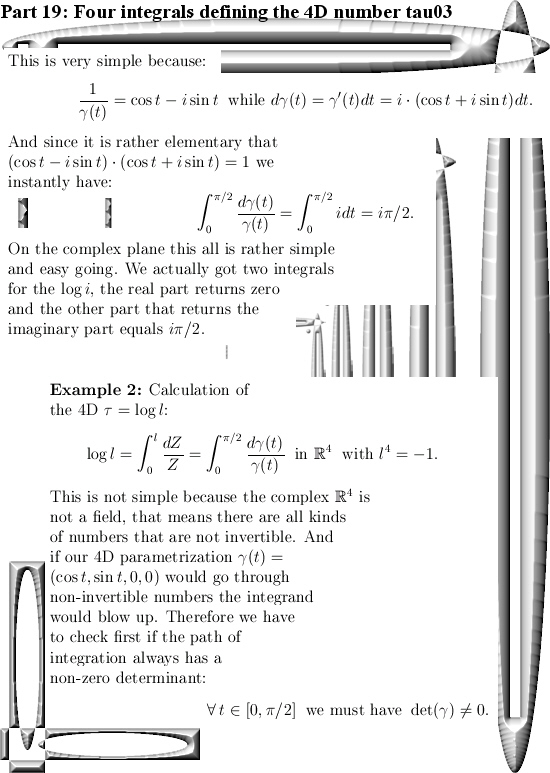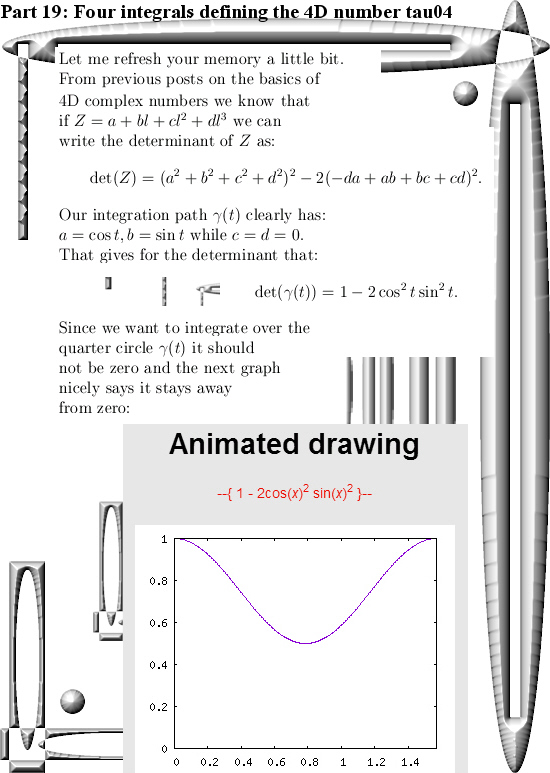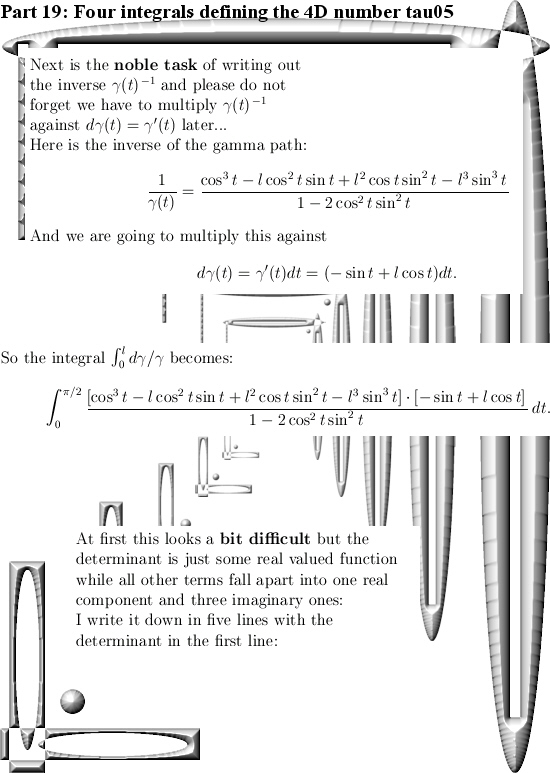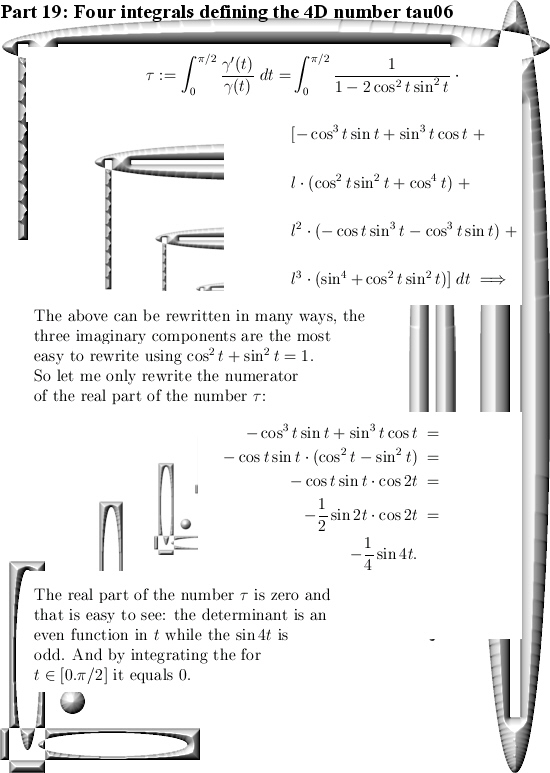End of this post.

Just a short video on the Fourier stuff.

This is the shortest post ever written on this website.

I found one of those video’s where the Fourier series is explained as the summation of a bunch of circles. Likely when you visit a website like this one, you already know how to craft a Fourier series of some real valued function on a finite domain.

You can enjoy a perfect visualization of that in the video below:

Only one small screen shot from the video: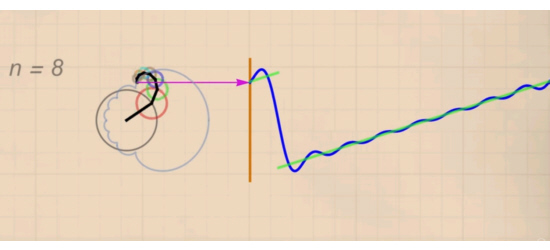Oh oh, the word count counter says 80+ words. Let me stop typing silly words because that would destroy my goal of the ‘shortest post ever’. Till updates.

Part 14: The Cauchy integral representation for the 4D complex numbers.

It took me longer than expected to craft this update. That is also the nature of the subject; you can view and do math with Cauchy integral representation in many ways. In the end I settled on doing it just for polynomials of finite degree and even more simple: these polynomials are real valued on the real line. (So they have only real coefficients and after that are extended to the space of 4D complex numbers).

In another development, last week we had the yearly circus of Noble prizes and definitely the most cute thing ever was those evolving protein molecules. Because if you can use stuff like the e-coli bacteria you can indeed try if you can (forcefully) evolve the proteins they make… That was like WOW. Later I observed an interview with that chemistry Nobel prize winner and she stated that when she began she was told ‘gentlemen don’t do this kind of thing’.

So she neglected that ‘gentlemen stuff’ and just went on with it. That is a wise thing because if you only do what all those middle age men tell you to do you will find yourself in the very same hole as they are in…
The physics prize was also interesting, for myself speaking I was glad we did not observe those physics men totally not understanding electron spin but with the usual flair of total arrogance keep on talking about spin up and spin down.

You can also turn that spin nonsense upside down: If elementary particles only carry monopole electrical charge than why should electrons be bipolar when it comes to magnetism? That Gauss law of magnetism is only a thing for macroscopic things, there is no experimental proof it holds for quantum particles…

But let’s talk math because this update is not about what I think of electron spin. This is the second Cauchy integral representation I crafted in my life. Now the last years I produced a whole lot of math, my main file is now about 600 pages long. But only that very first Cauchy integral representation is something that I printed out on a beautiful glossy paper of size A0. That first Cauchy integral representation was on the space of 3D numbers and there life is hard: The number tau has determinant zero and as such it is not invertible. But I was able to complexify the 3D circular numbers and it was stunning to understand the number tau in that complexification of the 3D circular numbers. Just stunning…

Therefore I took so much time in trying to find an easy class of functions on the space of 4D complex numbers. I settled for easy to understand polynomials, after all any polynomial gives the same value everywhere if you write them as a Taylor series.
Since this property of polynomials is widely spread I can safely say this in this part 14 of the basics to the 4D complex numbers we have the next Theorem:
THEOREM: The math will do the talking.
PROOF: Just read the next 12 pictures. QED.

As usual all pictures are 550 x 775 pixels in size. I also use a thing I name ‘the heart of the Cauchy integral’, that is not a widely known thing so take your time so that the mathematical parts of your brain can digest it…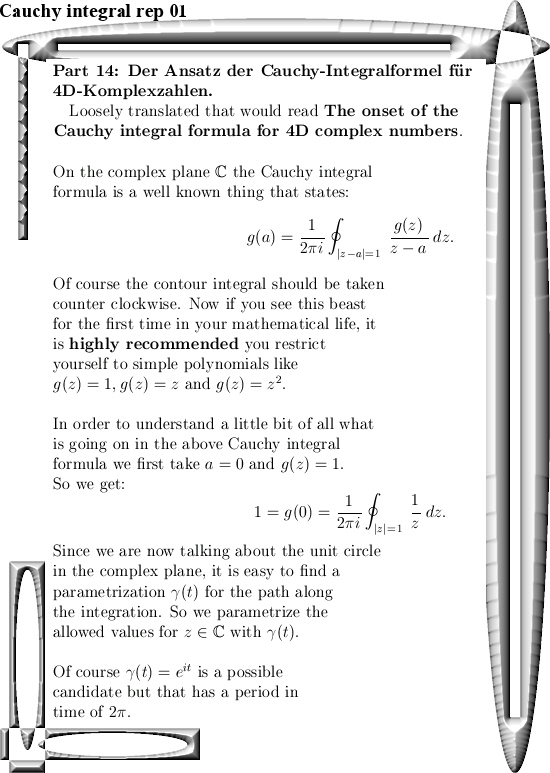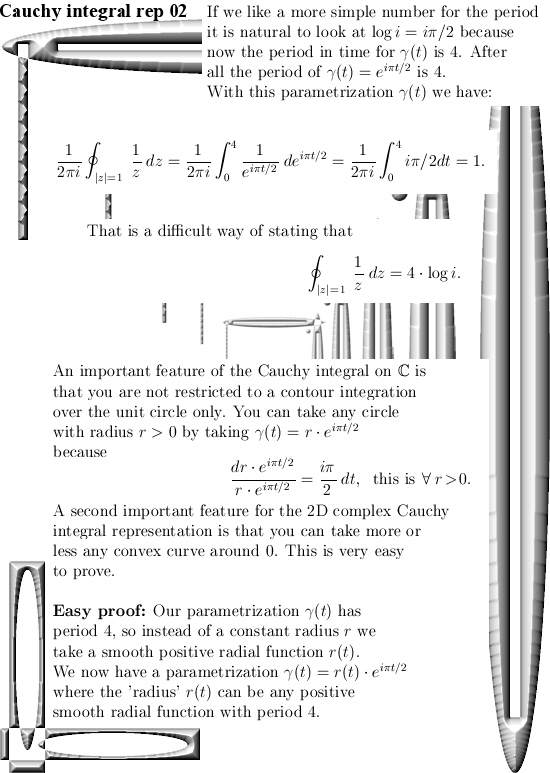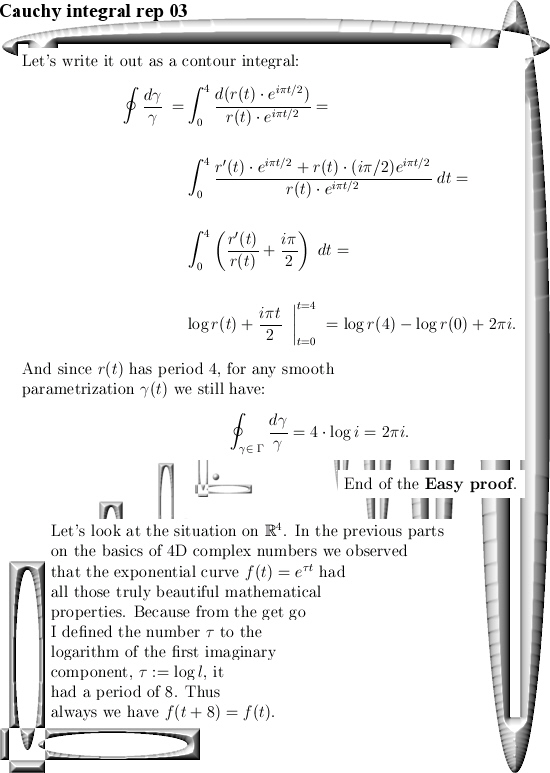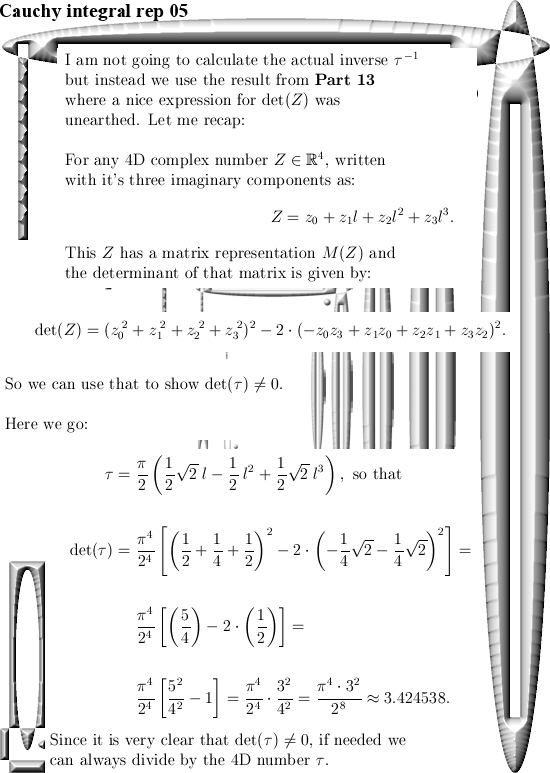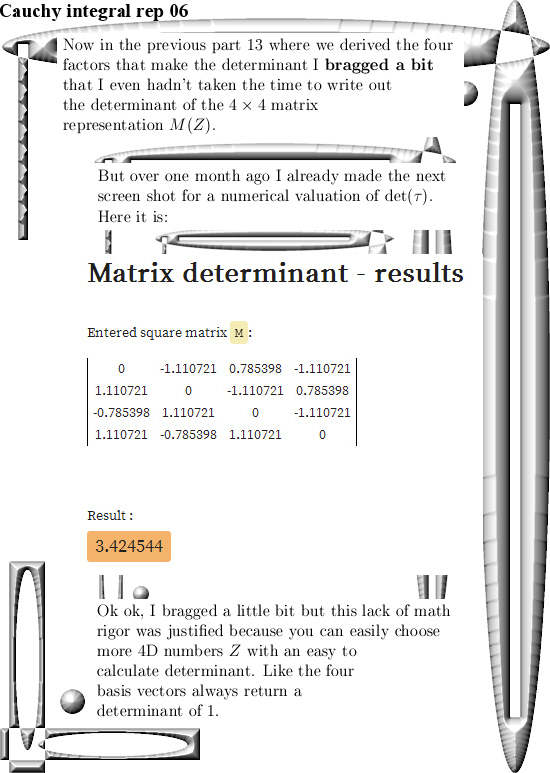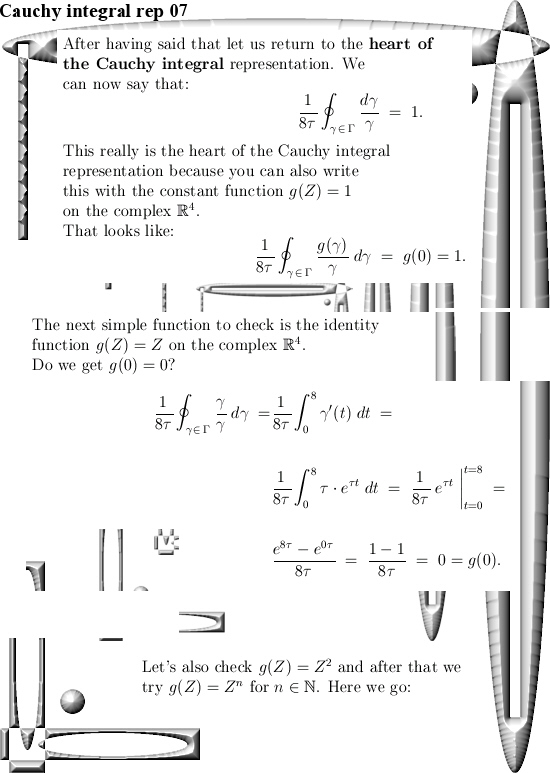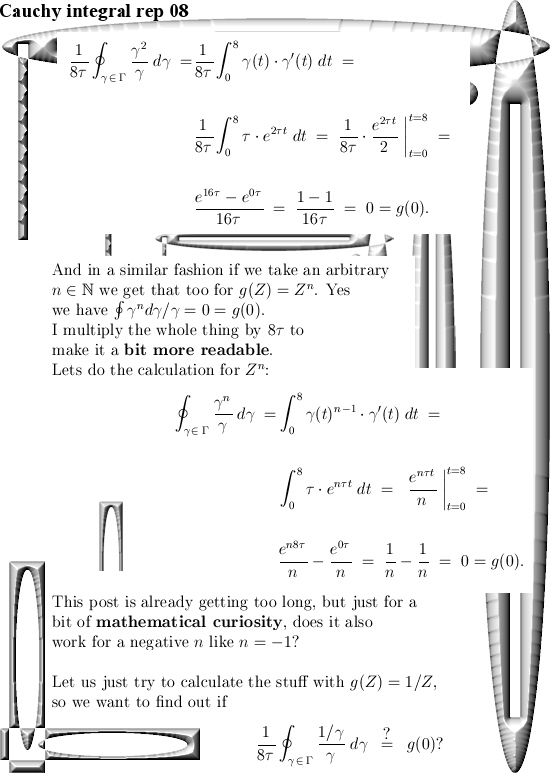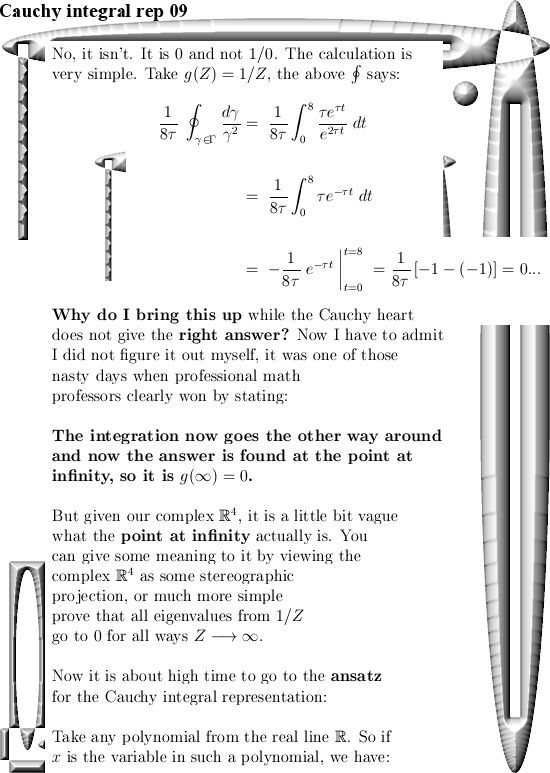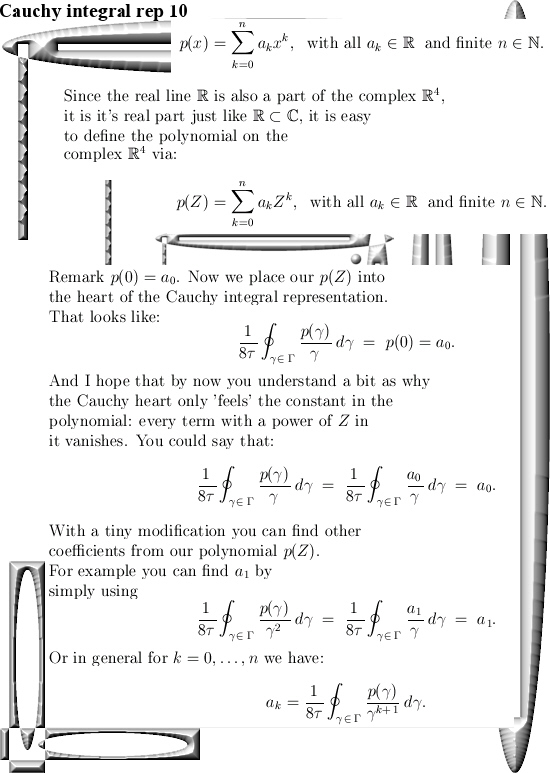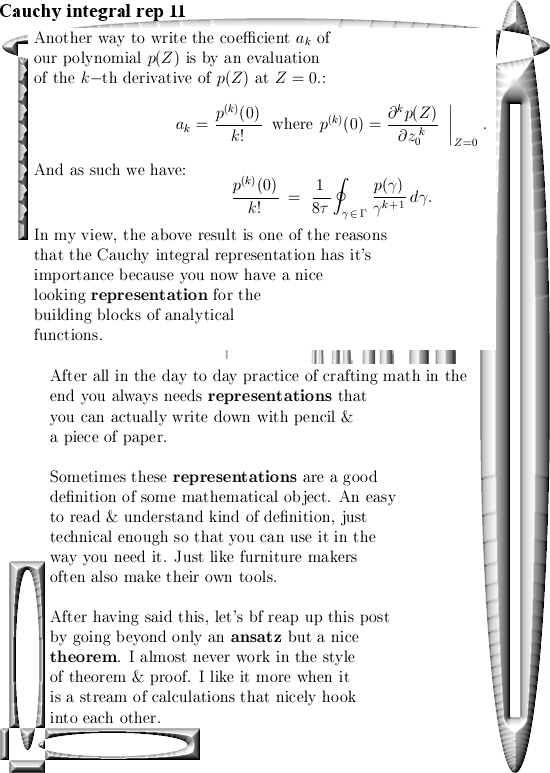I truly hope the math in this update was shallow enough so you can use it in your own path of the math that you like to explore.

End of this post, may be in Part 15 we will finally do a bit more about the diagonalization of 4D complex numbers because that is also a universal way of finding those numbers tau in the different dimensions like the 17D circular numbers & all those other spaces.

Have a nice life or try to get one.

A few numerical results related to the 4D sphere-cone equations using the four coordinate functions of the 4D exponential curve.

This is Part 9 in the basics to the complex 4D numbers. In this post we will check numerically that the 4D exponential curve has it’s values on the 4D unit sphere intersected with a 4D cone that includes all coordinate axes. In 3D space the sphere-cone equations ensure the solution is 1 dimensional like a curve should be. In 4D space the sphere-cone equations are not enough, there is at least one missing equation and those missing equations can be found in the determinant of a matrix representation M(Z) for a 4D complex number Z.

But we haven’t done any determinant stuff yet (because you also need a factorization of the 4D determinant in four variables and that is not a trivial task). So this post does not contain numerical evidence that the determinant is always one on the entire exponential curve.

If you want to compare this post to the same stuff in the complex plane:
In the complex plane the sphere-cone equation is given by x^2 + y^1 = 1 (that is the unit circle) and if you read this you probably know that f(t) = e^{it} = cos t + i sin t.

You can numerically check this by adding the squares of the sine and cosine for all t in one period and that is all we do in this post. Only it is in 4D space and not in the two dimensional complex plane…

This post is seven pictures long (all of the usual size 550 x 775).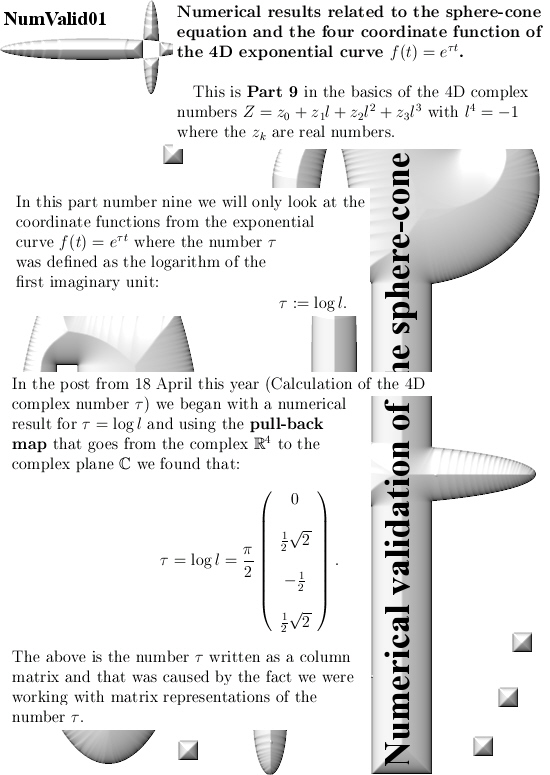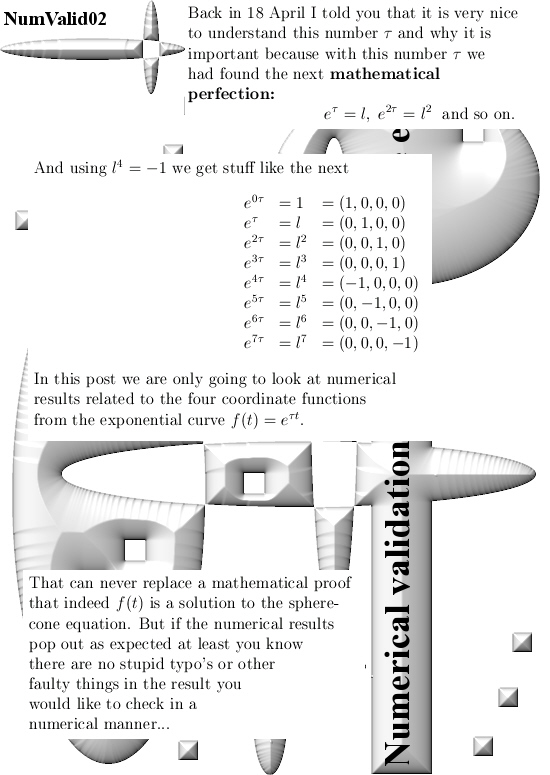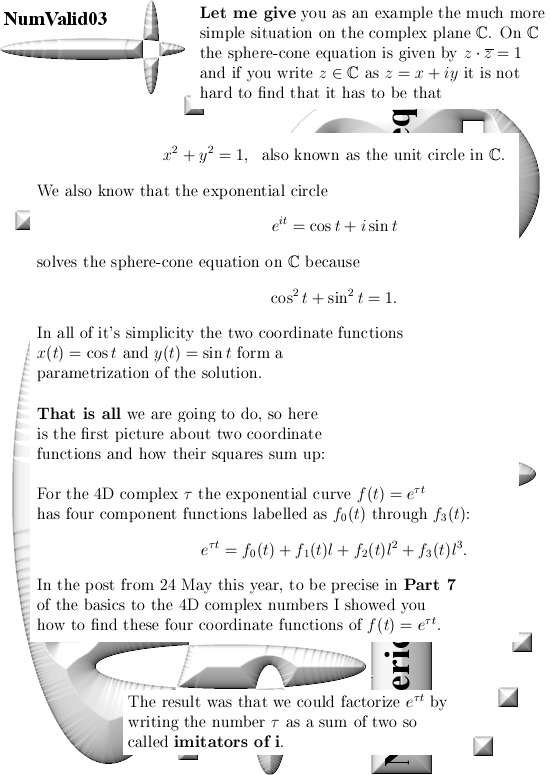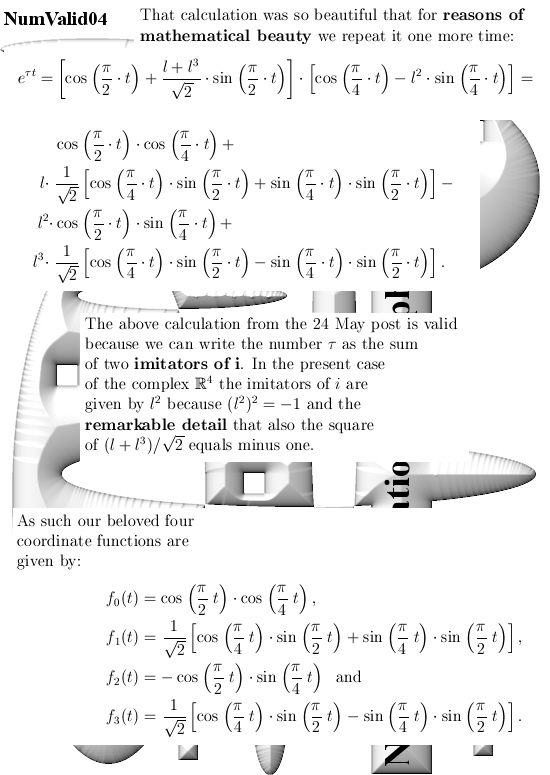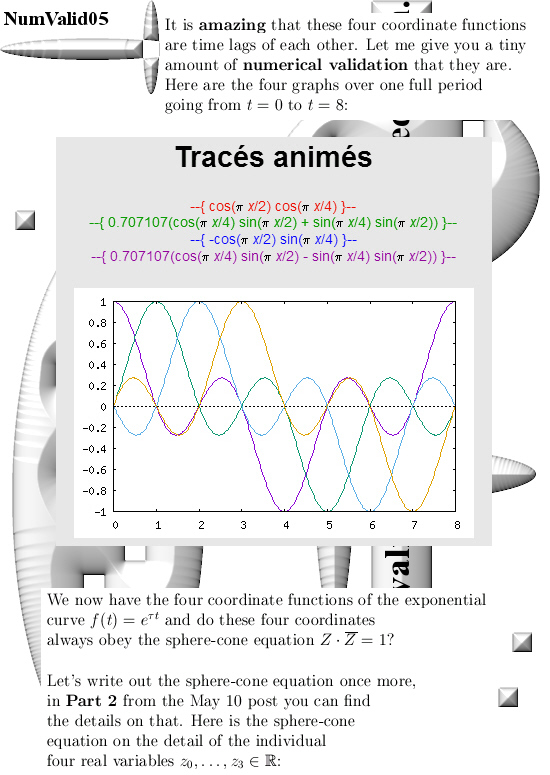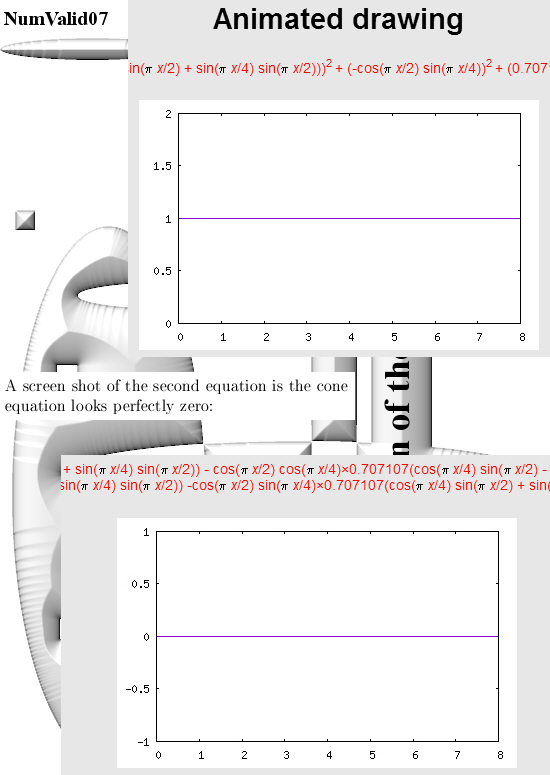All graphs in this post are made with the applets as found on:

For the two graphs from above look for ‘animated drawing’ choose the 2D explicit curves option. There you must use the variable x instead of time t.

Here is the stuff you can place in for the sphere equation:

(cos(pi*x/2)*cos(pi*x/4))^2 +
(0.707107*(cos(pi*x/4)*sin(pi*x/2) + sin(pi*x/4)*sin(pi*x/2)))^2 +
(-cos(pi*x/2)*sin(pi*x/4))^2 +
(0.707107*(cos(pi*x/4)*sin(pi*x/2) – sin(pi*x/4)*sin(pi*x/2)))^2

If you just ‘cut & paste’ it should work fine…
That should save you some typo’s along the way

Ok, that is what I had to say on this numerical detail.

Calculation of the four coordinate functions for the 4D exponential curve (complex version).

Like promised in this post I will show you in the greatest detail possible how to find those rather difficult looking four coordinate functions.

I had thought about crafting these four coordinate functions before but the method I had in mind was rather labor some so I balked a bit at that. Not that I am lazy but I also had to work on the basics for the 4D complex numbers like in the last posts…

So one day I decided to look into the specific details of what I name ‘imitators of the number i’ and I was very surprised by their behavior. As a matter of fact these imitators imitate i soo good that you can make exponential circles of them.
And I wrote down the two exponential circles, I looked at them and realized you can factorize the 4D exponential curve with it and as such you will get the four coordinate functions…

That was all, at some point in time on some day I just decided to look at the imitators of the number i from the complex plane and within 5 at most 10 minutes I found a perfect way of calculating these four coordinate functions.

It always amazes me that often a particular calculation takes a short amount of time, like 10 or 20 minutes, and after that you always need hours and hours until you have a nice set of pictures explaining the calculation…

Anyway, this post is five pictures long and as such it contains also Part 6 and 7 of the Basics to the 4D complex numbers.

I hope that in the long run it will be the result in this post that will make 4D complex numbers acceptable to the main stream mathematical community.
But may be once more I am only fooling myself with that, after all back in the year 1991 I was only thinking stuff like ‘If you show them the 3D Cauchy-Riemann equations, they will jump in the air from joy’.
They (the math professors) never jumped from joy, no significant change in brain activity was ever observed by me. So when I write ‘in the long run’ as above, may be I should more think like a geological timescale…
__________

But let’s not complain because once you understand the factorization, it is so beautiful that it is hard to feel angry or whatever what.
Here are the five pictures: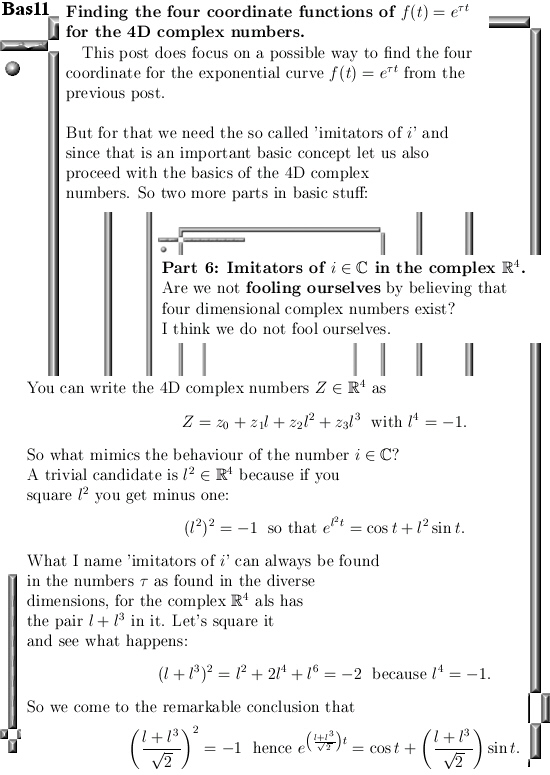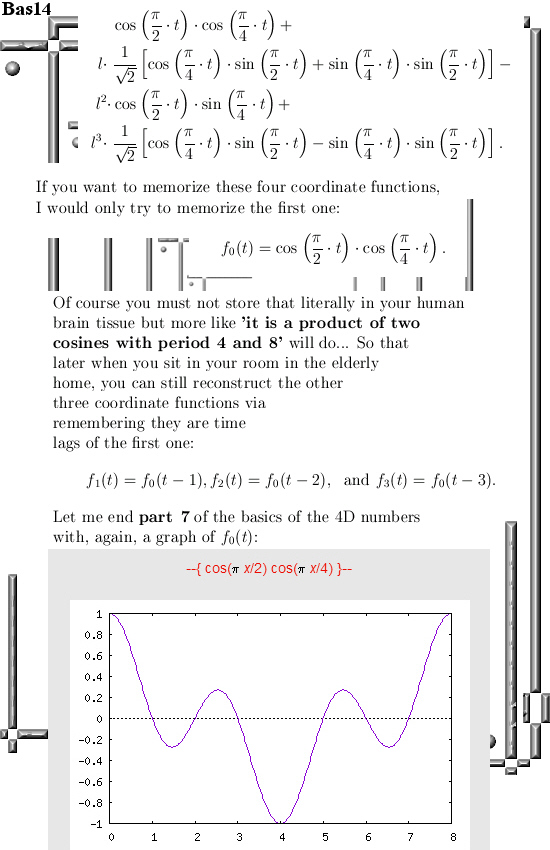Ok, that is how you calculate the four coordinate functions.

A nice teaser picture about 4D coordinate functions of the 4D exponential curve (complex version).

Lately I have been working on the next post about the basics of the 4D complex numbers. You simply need those basics like matrix representations because later on when you throw in some 4D Cauchy-Riemann equations, it is very handy to have a good matrix representation for the stuff involved.

The next post covering the basics had five parts, let’s not dive in all kinds of math details right now but go straight to part five with the four coordinate functions of the 4D exponential curve: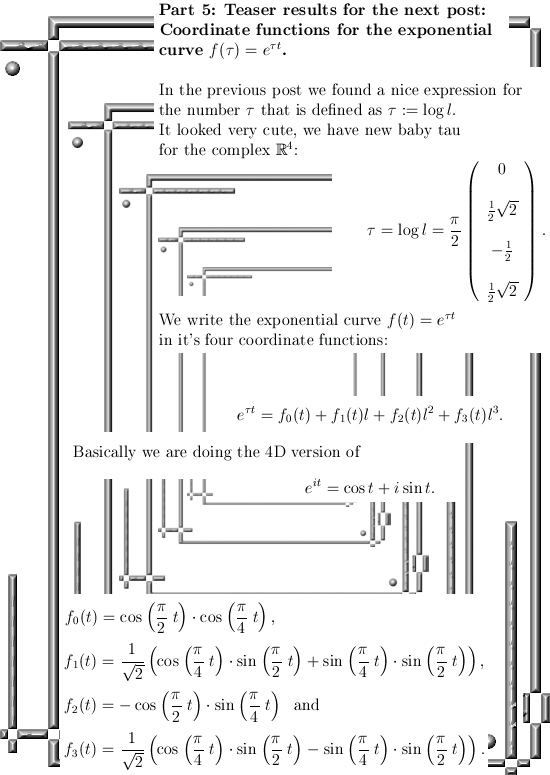These four coordinate functions are also time lags of each other.

This new baby number tau keeps on looking cute…

Let me leave it with that, till updates.

I am innocent, I did not do it. I just found the numbers tau in the Schrödinger equation your honour…

Judge: But you were caught red handed placing the number tau in a Schrödinger equation while you do not qualify for being a member of the most bright and enlightened persons in our society: The PHYSISCS PROFESSORS.

Reinko: But Judge I can explain, it was that evil guy that Gerard ‘t Hooft who did it. I can prove that because it is on video.

Judge: Yes you already told that into the statements you made after arrest by the police. So we took the freedom and ask Mr. Gerard ‘t Hooft himself about the evil you have done with molesting the Schödinger equation. Mr. ‘t Hoofd said it had to be Hermitian and although I do not know what that means he said that by using anti-Hermitian matrices you, Reinko Venema, you are nothing more as some sadistic pedophile piece of shit.

Reinko: But judge, it is not Hermitian, that is only a trick. You see if you multiply it by the number 1 like 1 = – i squared you see it is not Hermitian.

Judge: Do you think we get complex analysis in law school? We don’t, we asked some experts and all agreed that Gerard is right and you are wrong and right now rewarded by your own evil deeds to 75 years in prison in a maximum security facility.

__________

After this somewhat strange introduction I repeat I was innocent. I was just looking at a video of a guy that is just like me old and boring.

And that guy, Gerard ‘t Hooft, was able to give me three nice punches in the face.
That is what this post is about; Three punches in the face as delivered by Gerard.

It is the very first time I observe professional physics professors using the number tau while claiming the stuff has to be Hermitian to make any sense.

I was devastated because in my little world of mathematics it had to be anti Hermitian so at a first glimpse it looks like a simple shootout between Gerard and me: Only one can be right…

Let me first show you the Youtube video where right at the start Gerard succeeds to bring my small sack of human brain tissue into an exited state and after that I am rewarded with finding the number tau into the famous Schrödinger equation.

Let me also temper the enthousiasm a little bit because at present date 26 Feb in the year 2018 I only know of one example where three quantum states are rotated into each other:
That is the transport of the color charges as it is found on the quarks inside the proton and neutron…

Here is the video, after that the nine pictures that make up the mathematical core of this new post:

Gerard ‘t Hooft – How Quantum Mechanics Modifies the Space-Time of a Black Hole (QM90)

Let me spare you a discussion on the entire video but only look at what you can find on the very introduction as shown above because all of the three punches at my face are already found there.

Here are the nine pictures for this new post: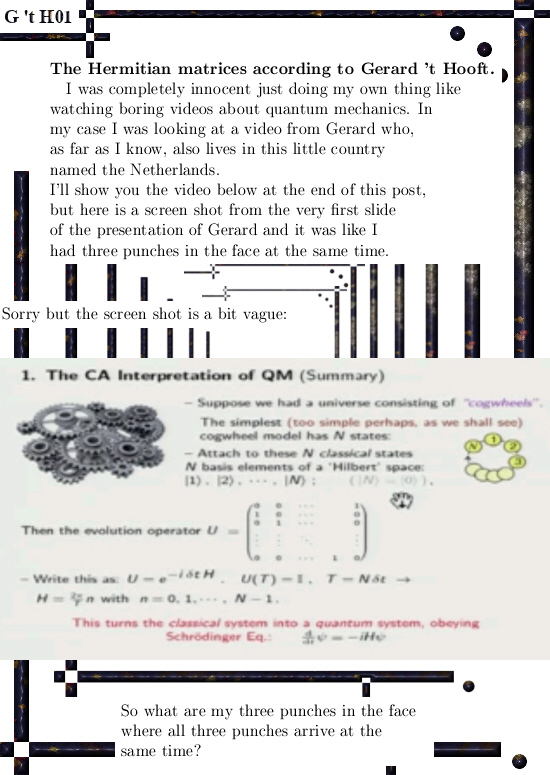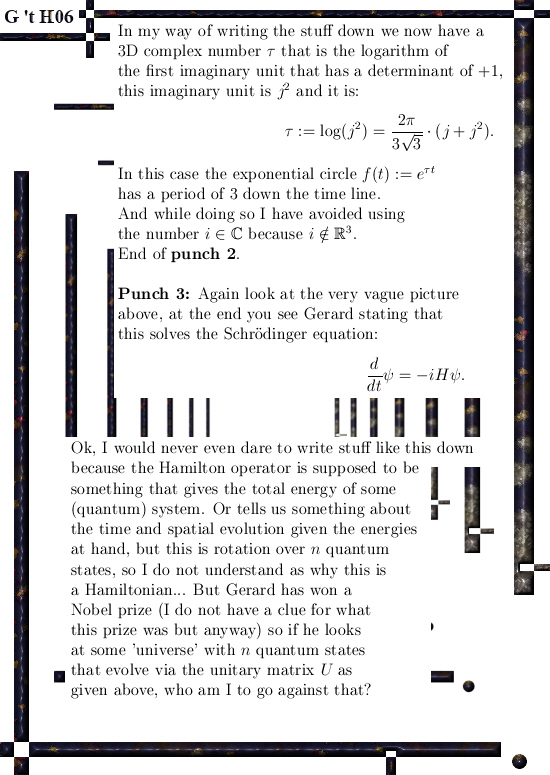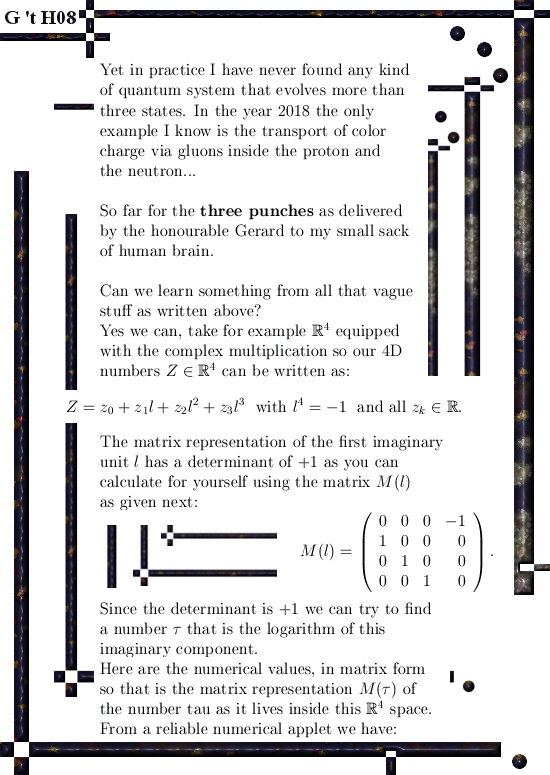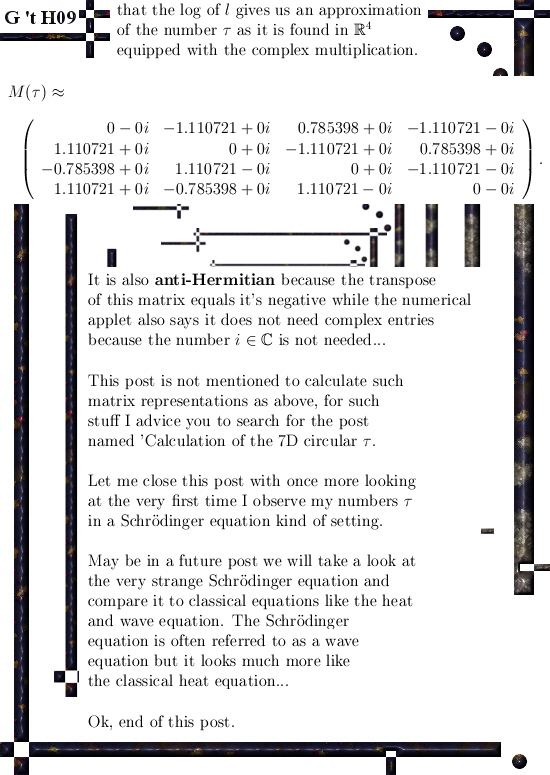For readers who have found themselves lost on what a Hermitian matrix is, here is a wiki:

Hermitian matrix
https://en.wikipedia.org/wiki/Hermitian_matrix

And for readers who have found themselves lost on finding an ‘analytic handle’ about how to calculate matrices like in picture 09, a good starter would be about the calculation of the 7D number tau:

An important calculation of the 7D number tau (circular version).

That’s it, till updates.